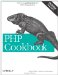# Recipe 6.8. Skipping Selected Return Values

#### 6.8.1. Problem

A function returns multiple values, but you only care about some of them.

#### 6.8.2. Solution

Omit variables inside of list( ):

`// Only care about minutes function time_parts(\$time) {     return explode(':', \$time); } list(, \$minute,) = time_parts('12:34:56');`

#### 6.8.3. Discussion

Even though it looks like there's a mistake in the code, the code in the Solution is valid PHP. This is most frequently seen when a programmer is iterating through an array using each( ), but cares only about the array values:

`while (list(,\$value) = each(\$array)) {     process(\$value); }`

However, this is more clearly written using foreach:

`foreach (\$array as \$value) {     process(\$value); }`

To reduce confusion, don't use this feature; but if a function returns many values, and you only want one or two of them, this technique can come in handy. One example of this case is if you read in fields using fgetcsv( ), which returns an array holding the fields from the line. In that case, you can use the following:

`while (\$fields = fgetcsv(\$fh, 4096)) {     print \$fields . "\n";  // the third field }`

If it's an internally written function and not built-in, you could also make the returning array have string keys, because it's hard to remember, for example, that array element 2 is associated with 'rank':

`while (\$fields = read_fields(\$filename)) {     \$rank = \$fields['rank']; // the third field is now called rank     print "\$rank\n"; }`

However, here's the most efficient method:

`while (list(,,\$rank,,) = fgetcsv(\$fh, 4096)) {     print "\$rank\n";         // directly assign \$rank }`

Be careful you don't miscount the amount of commas; you'll end up with a bug.

Recipe 1.11 for more on reading files using fgetcsv( ).PHP Cookbook: Solutions and Examples for PHP Programmers
ISBN: 0596101015
EAN: 2147483647
Year: 2006
Pages: 445

Similar book on Amazon